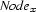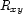var2node method

Whenever, in a literal, we encounter an atom naming a variable, we invoke method var2node to make sure that this variable is already encoded and to retrieve the corresponding node representation. If this is the first time we encounter this variable, we allocate for it a new integer and create a node representationfor it. Further, we also createfor all variablesthat were known sofar (i.e. all variables encoded by integers smaller than the one encoding).

meth var2node(X Node)
I = {Dictionary.condGet @var2int X unit}
in
if I==unit then
I=(counter<-I)+1
in
{FS.include I @vars}
Node={MakeNode I @vars}
@var2int.X  := I
@int2node.I := Node
for J in 1..(I-1) do
@choices.(I*1000+J) := {FD.int 1#4}
end
else Node=@int2node.end
end

Denys Duchier
Version 1.2.0 (20010221)